# Machine

(in mathematics)

An abstract device realizing information processing. The terms "abstract machineabstract machine" and "automatonautomaton" are also used. Abstract machines are particular cases of control systems (cf. Control system). They arose in connection with the analysis of the concept of an algorithm, starting in the middle of the 1930s, with the development of computers and with the construction of mathematical models of biological systems. Machines processing discrete information became most widespread. Typical representatives of these are finite automata (cf. Automaton, finite) and Turing machines (cf. Turing machine). Abstract machines are easily visualized, they can be easily combined in various ways, and they have simple operating steps. Machines are being studied within the frame of the theory of algorithms (cf. Algorithms, theory of) and mathematical cybernetics and their study aims at an analysis and formalization of the concept of an algorithm and at modelling real devices and processes in a mathematical way. There is a fruitful relation between abstract machines and real computers. The ideas of constructing computers and of programming them relies, to a large extent, on ideas from the theory of algorithms and mathematical cybernetics. In turn, practical work with computers poses new problems and suggests models of machines in order to solve these problems.

Common to all abstract machines is the presence of a finite control device that may be in one of the states. A machine has a potentially-unbounded exterior memory and has a means for reading and writing information into the exterior memory. Reading (writing) of information is, as a rule, carried out locally. The operation of a machine is determined by a program, consisting of commands of the form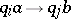, where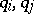are states of the control device,stands for ordered information of local character from the exterior memory andis the notation for both the change of the contents of the exterior memory and the position of the reading device in it. The machine operates in discrete time. At each stepthe machine uses the reading device to move informationfrom the exterior memory to the control device. If at stepthe control device of the machine is in stateand the program contains the command, then at the next step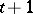the control device is in state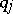, and the contents of the exterior memory and the position of the reading device are changed according to. Usually one distinguishes one or several initial or final states among the states of the control device. Before starting to operate, the control device is in an initial state, and the end of the operation is determined by the final state(s).

In many cases an abstract machine is constructed to compute numerical (word) functions and predicates. The computation of a function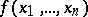on a machineis understood as follows. For an arbitrary choice of the arguments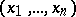an initial configurationofis indicated, this being a filling of the external memory and a position of the reading device with respect to it corresponding to. It is determined which configurations ofare final, i.e. when the computation ofonis terminated. It is determined what value of the function to be computed is obtained for each final configuration. The computation of the value ofconsists of a series of transitions from the initial state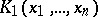to the next state, etc., in correspondence with the program of the machine, until a final configuration is reached. If the value ofis not defined,will never reach a final configuration when starting from. In this case the computing process may continue indefinitely.

Often one uses an ordinary Turing machine in the definition of an abstract machine; the differences consist of adding new possibilities or restrictions on the Turing machine. The modification of a Turing machine is usually conducted along the following three lines.

## 1. The organization of the exterior memory.

The introduction of several tapes is most common (multi-tape Turing machines). One also studies machines with one-sided or multi-dimensional tapes, with an exterior memory in the form of an infinite graph (e.g. in the form of an infinite binary tree). Another approach consists in replacing tapes by registers or calculating frames, suitable for containing natural (integral) numbers or words of arbitrary length. Such are Shepherdson–Sturgis machines , the Minski machine  and a random-access machine.

## 2. The storage, transformation and obtaining of information from the external memory.

One considers machines for which a part of the information in the external memory to which the control device has had no access for some time (depending on the input) becomes totally inaccessible to the reading device for the rest of the time. The definition of a Turing machine with tapes of storage type is based on a closely related idea (pushdown automata).

For machines taking and transforming information from the external memory symbol-by-symbol, a typical restriction is the prohibition to print some symbols next to certain others. Such are, for example, the non-erasing Turing machines and two-sided automata equivalent to finite automata. The important class of finite automata also belongs here. However, restrictions on the size of the memory, the operating time, etc., are more usual. For example, a linearly bounded automaton is a Turing machine for which the length of the tape used in the computations is bounded by a linear function in the length of the input.

Often, the information from the external memory is obtained by using several reading heads. A more usual approach is where general recursive predicates have been defined on the external memory. The set of these predicates determines the information arriving at the control device of the machine. This idea is used in Shepherdson–Sturgis machines, where the addition of information into the external memory is realized by arbitrary general recursive functions.

## 3. The means of functioning.

Here one distinguishes between deterministic, non-deterministic and probabilistic machines. For a deterministic machine every operating step is uniquely determined by the state of the control device and the information from the external memory obtained by the reading device. The program of a non-deterministic machine may contain different commands with the same left-hand side. Therefore, for a non-deterministic machine one considers not one but the set of all computations compatible with the program for a given input. A probabilistic machine is a machine that is provided with a random-number generator or one that has a program in which the transition from one command to another is realized with a given probability.

In the non-deterministic case one usually considers the computation of predicates. If a non-deterministic machinecomputes a predicate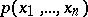, thenis true if and only if among all possible computations ofstarting at the configurationthere is a computation containing a final configuration.

In general, the computing possibilities of deterministic, non-deterministic and probabilistic machines are the same. However, in separate narrow classes of machines, non-deterministic and probabilistic machines may prove to be more powerful than deterministic machines. For many types of abstract machines with restrictions either on the volume of the external memory or on the operating time, the problem of "determinization" of non-deterministic machines is an interesting open (1989) problem.